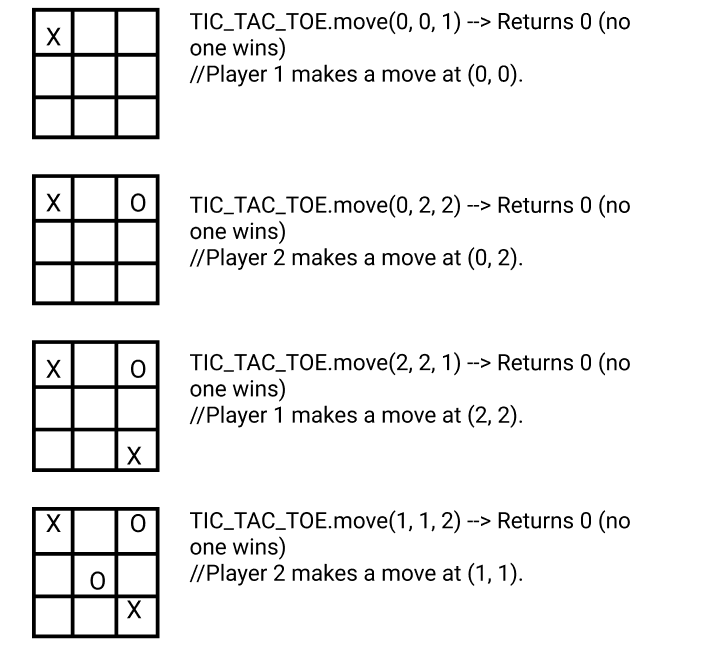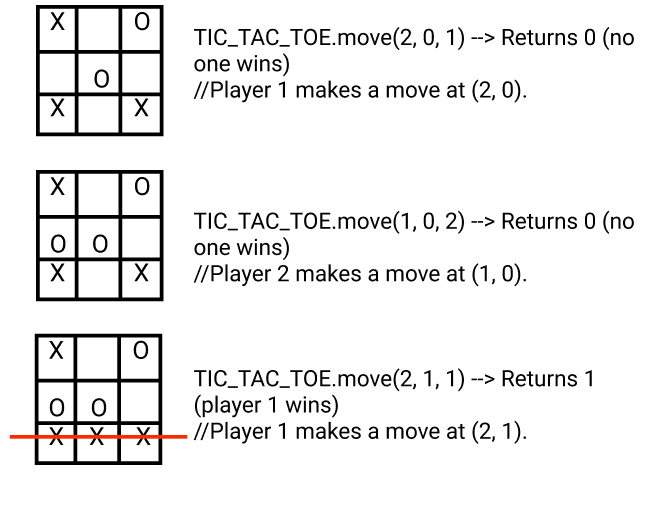New update is available. Click here to update.

# Design Tic-Tac-Toe

Last Updated: 20 Mar, 2021
Difficulty: Moderate

## PROBLEM STATEMENT

#### Ninja has to assume the following rules while playing the game:

``````This game is played between two people (Player 1 and Player 2). Player 1 chooses ‘X' and Player 2 chooses ‘O’ to mark their cells. A move is guaranteed to be valid and is placed on an empty block.

A player who successfully places 'N' of their marks in a horizontal, vertical, or diagonal row wins the game. Once a winning condition is reached, no more moves are allowed.
``````

#### The function must return an integer which is either 0, 1, and 2 representing:

``````0: No one wins.
1: Player 1 wins.
2: Player 2 wins.
``````

#### Example:

``````Let us assume if ‘N’ = 3 and player 1 places ‘X’ and player 2 places ‘O’ on the board.
``````##### Input Format
``````The first line of input contains an integer ‘T’ which denotes the number of test cases.

The first line of each test case contains an integer ‘N’ that represents the size of the ‘N’ * ‘N’ grid.

The next line of each test case contains an integer ‘Q’ that represents the number of queries.

The next ‘Q’ lines of each test case contain three single space-separated integers ‘ROW’, ‘COL’, and ‘PLAYER’ representing the current row and column of the grid and the current player (1 or 2).
``````
##### Output Format :
``````For each test case, Ninja has to complete the function ‘move’ and return either 0, 1, and 2.

Print the output of each test case in a separate line.
``````

#### Note:

``````You do not need to print anything; it has already been taken care of. Just implement the given function.
``````
##### Constraints:
``````1 <= ‘T’ <= 100
1 <= ‘N’ <= 100
5 <= ‘Q’ <= ‘N*N’
0 <= ‘ROW’ , ‘COL’ < ‘N’
‘PLAYER’ = {1, 2}

Where ‘ROW’ and ‘COL’ represent the current row and column of the grid and ‘PLAYER’ represents the current player.

Time Limit: 1 sec
``````